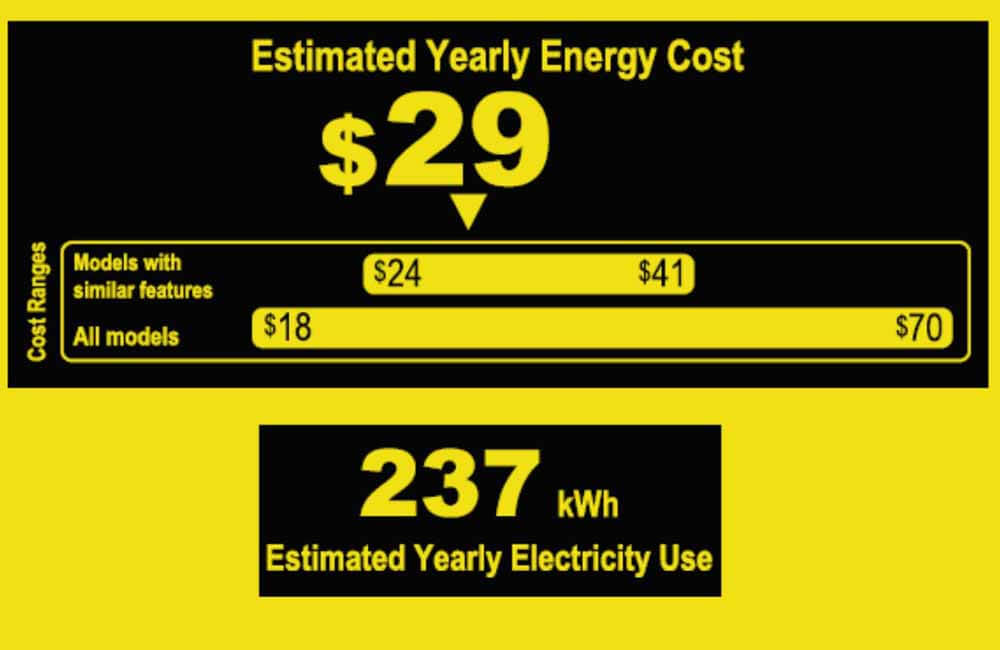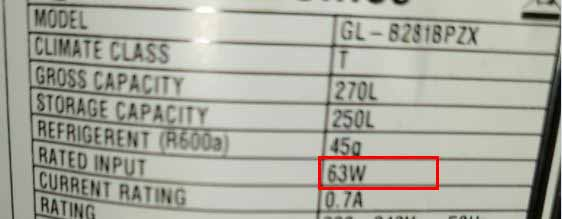# How much electricity does a mini fridge use? Everything you need to know

The amount of electricity a mini fridge uses depends on a few factors, such as ambient temperature, insulation, usage, age and model of the fridge, etc…

However, in general, mini fridges consume between 200 and 280 kWh (kilo-Watt-hours) of energy per year.In this article, I will put this electricity consumption into perspective by comparing different types of refrigerators. And I will show you how you can estimate your own mini fridge’s electricity consumption.

Page Contents:

## How much electricity does a mini fridge use?

On average, a mini fridge uses 65 watts of power when it’s running, and runs for 8 to 10 hours per day. This translates to about 600 Wh of daily energy consumption, and 220 kWh of yearly energy consumption.

At 12 cents per kWh (kilo-Watt-Hour), it would cost \$20 to \$30 per year to run a mini fridge.

Here are a few examples of some mini fridges, their sizes, their power consumption, and how much it would cost to run each of them.

If you want to estimate how much electricity your mini-fridge uses, make sure not to confuse electrical power and electrical energy.

• Electrical Power is the product of voltage and current and is measured in Watts.
• Electrical Energy is the product of electrical power and usage time and is measured in Watt-hours.

### How much power does a mini fridge use?

Depending on the model and the size, a mini fridge uses between 60 and 80 Watts when it’s running, and about 200 Watts when it’s starting (for a couple of seconds).

However, older mini fridges may require more power to run.

Just like other types of refrigerators, a mini fridge’s wattage is usually mentioned on its specification label.You can determine how much power a mini fridge uses by checking its spec sheet or by googling the model number.

The wattage of a mini fridge is very useful when trying to estimate how much energy it consumes.

More on that below.

### How much energy does a mini fridge use?

On average, a mini fridge consumes about 600 Wh of energy per day. Which means a monthly consumption of 18 kWh and a yearly consumption of about 220 kWh.

Most mini fridges are EnergyStar rated, and come with an EnergyGuide label that estimates their yearly energy consumption. Such as this one:The EnergyGuide label above is from a Kenmore 99059 4.5 Cu. ft. mini-fridge, and it estimates that the fridge’s yearly electricity use is about 237 kWh.

It also estimates how much it would cost to run, and even compares it to other models. Which is pretty useful, especially if you want to run a refrigerator on solar power.

If for some reason your mini fridge does not have an EnergyGuide label, you can still estimate its energy consumption using its Wattage.

As mentioned above, electrical energy is the product of electrical power and usage time:

Electrical Energy (Watt-hours) = Electrical power (Watts) x Usage time (hours)

For example:

I have a desk lamp that’s rated at 40 watts; if I leave this lamp on for 2 hours (usage time), it will consume 80 Watt-hours of energy.

It’s the same with mini fridges. Except that when a mini fridge is plugged in, it’s not technically ON all the time.

As a general rule of thumb, it’s only ON for one-third of the time. So for every 24 hours, the compressor in a mini fridge only runs for about 8 hours.

For example:

I have a mini fridge that’s rated at 63 Watts; it’s estimated daily energy consumption can be calculated as such:

Estimated Daily Energy Consumption (Wh) = Wattage (Watts) x 8 hours

Estimated Daily Energy Consumption (Wh) = 63 Watts x 8 hours

Estimated Daily Energy Consumption (Wh) = 504 Watt-hours

## Do mini fridges use a lot of electricity?

On average, mini-fridges consume about 240 kWh of energy per year. Which amounts to about \$29 per year in electricity bills.

To put this into perspective, the table below compares the electricity usage of different types of refrigerators:

With an average energy consumption of 600Wh per day, mini-fridges consume 3 to 4 times less energy than regular size refrigerators.

But does that mean that a mini fridge is more energy-efficient than a full-size fridge?

If we compare the energy consumption per cubic foot of a full-size fridge (about 75Wh/ft3 per day) to that of a mini-fridge (about 150 Wh/ft3 per day), full-size refrigerators seem to be more energy-efficient.

So if you’re thinking about getting 2 mini fridges instead of a full-size refrigerator, that might not be such a good idea.

But even though they consume more energy per cubic foot, mini fridges are quite efficient if you only need 1.7 to 4.5 cubic feet of space.

## FAQs:

### How many amps does a mini fridge use?

On average, a standard 120VAC mini fridge draws between 0.5A and 1A when it’s running, and can draw up to 3 Amps when it’s starting.

### How much solar power to run a mini fridge?

To run a mini fridge on solar power, you would need a solar array that produces about 600Wh (Watt-hours) of energy per day.

In other words, you would need 100 to 200 watts of solar power to run a mini fridge. The more sunlight your receive in your area, the less solar power you need.

For example:

To run a mini-fridge on solar panels, someone in Denver, Co would only need 100 Watts of solar power, while a person in Chicago, IL would require 200 watts.

For more information please refer to this article: How much solar power do I need to run a refrigerator

### What kind of solar system do you need to run a mini fridge?

To run a mini fridge on solar, you would need a solar system that consists of:

### How much solar for a 12V refrigerator?

A common 12V refrigerator (1.7 Cu. ft.) consumes about 400Wh (watt-hours) of energy per day. To power a 12V refrigerator only on solar power you would need a 100W solar panel.

### How much battery does a 12V refrigerator use?

A 12V refrigerator connected to a 12V car battery (50Ah) would be able to run for 1 to 2 days before the battery is completely depleted.

If connected to a 12V-100Ah battery, it could run for 3 to 4 days (depending on temperature, insulation, etc…).

Spread knowledge... It's FREE!!##### Younes

Hi! My name is Younes. I'm an electrical engineer and a renewable energy enthusiast. I created renewablewise.com with a mission of delivering digestible content and information to the people who seek it.